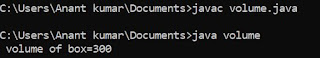## Saturday, 4 August 2018

### Program To Find Maximum Of Three Number Using 'If else' Statement

/*program to find maximum of 3 number*/
#include <stdio.h>
void main ()
{
int a,b,c;
clrscr ();
printf ("/n Enter value of a :");
scanf ("%d", & a);
printf ("/n Enter value of b :");
scanf ("%d", & b);
printf ("/n Enter value of c :");
scanf ("%d", & c);
if ((a>>b)&&(a>>c))
printf ("/n a is maximum number:");
else if ((b>>a)&&(b>>c))
printf ("/n b is maximum number:");
else
printf ("/n c is maximum number:");
getch ();
}

### OUTPUT :

Enter value of a : 45
Enter value of b : 55
Enter value of c : 65

65 is the greatest number.

ALGORITHM :: MAXIMUM OF THREE NUMBER ( A , B AND C )

This algorithm is used to determined the maximum of three number say ( a , b , c )

STEP 1. START.
STEP 2. INPUT A , B AND C.
STEP 3. [ MAXIMUM OF THREE NUMBER A , B AND C ]

1. IF A IS GREATER THAN B AND A IS ALSO GREATER THAN C.
2. PRINT " A IS MAXIMUM ".
3. IF B IS GREATER THAN A AND B IS ALSO GREATER THAN C.
4. PRINT " B IS MAXIMUM ".
5. IF C IS GREATER THAN A AND C IS ALSO GREATER THAN B.
6. PRINT " C IS MAXIMUM ".

[ END OF LOOP 3 ]

STEP 4. STOP